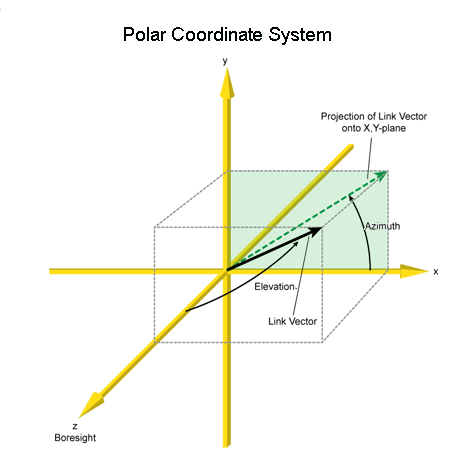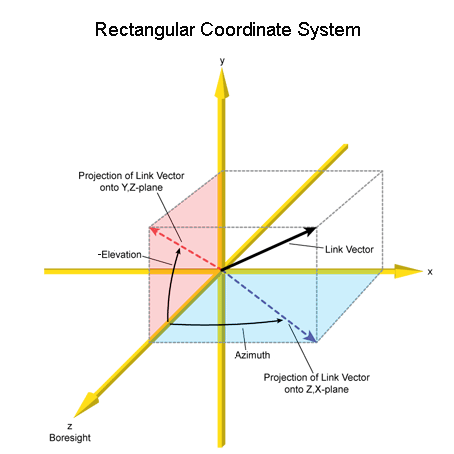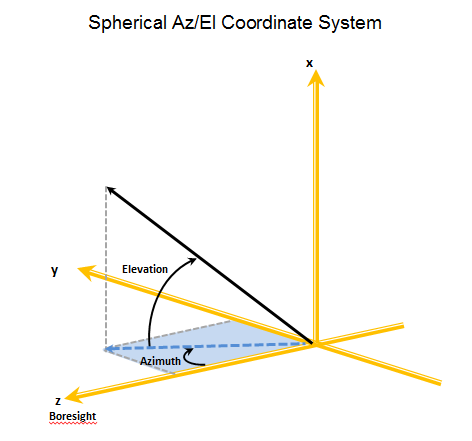# Antenna Coordinate Systems

Each of the antenna models in STK uses one of three coordinate systems to define the directional gain pattern:

Each system is defined by two angles, azimuth and elevation, that are measured relative to the antenna body axes.

The antenna boresight for all coordinate systems corresponds to the +z axis.

## Polar Coordinate System

Azimuth: The azimuth angle is defined as the clock angle about the positive z axis, starting at the positive x axis toward the positive y axis. The azimuth angle can range from -180 degrees to +180 degrees.

Elevation: The elevation angle is defined as the cone angle between the direction of interest and the antenna body z-axis. The elevation angle can range from 0 degrees to 180 degrees. An elevation angle of 0 degrees means the direction aligns with the positive z axis. An elevation angle of 180 degrees means that the direction aligns with the negative z axis.## Rectangular Coordinate System

This coordinate system is traditionally used by geo-stationary satellites where azimuth is from the boresight (z axis) toward the east, and elevation is from the equator to the north.

Azimuth. The azimuth angle is defined as the angle between the projection of the direction vector onto the zx plane and the z axis. Positive azimuth is measured toward the positive x axis. The azimuth angle can range from -180 degrees to 180 degrees.

Elevation. The elevation angle is defined as the angle between the projection of the direction vector onto the zy plane and the z axis. Positive elevation is toward the negative y axis, and negative elevation is toward the positive y axis. The elevation angle can range from -180 degrees to 180 degrees.## Spherical Az/El Coordinate System

Azimuth. The azimuth angle is defined as the angle measured from the z axis to the projection of the direction vector into the zy plane. The azimuth angle can range from -180 to 180 degrees. Positive azimuth is the z axis toward the positive y axis and negative azimuth is from the z axis toward the negative y axis.

Elevation. Elevation is defined as the angle between the zy plane and the direction vector. The elevation angle can range from -90 degrees to 90 degrees. Positive elevation is toward the positive x axis.